Ch.15 - Acid and Base EquilibriumWorksheetSee all chapters
 Ch.1 - Intro to General Chemistry 2hrs & 53mins 0% complete Worksheet Ch.2 - Atoms & Elements 2hrs & 49mins 0% complete Worksheet Ch.3 - Chemical Reactions 3hrs & 25mins 0% complete Worksheet BONUS: Lab Techniques and Procedures 1hr & 38mins 0% complete Worksheet BONUS: Mathematical Operations and Functions 47mins 0% complete Worksheet Ch.4 - Chemical Quantities & Aqueous Reactions 3hrs & 55mins 0% complete Worksheet Ch.5 - Gases 3hrs & 47mins 0% complete Worksheet Ch.6 - Thermochemistry 2hrs & 28mins 0% complete Worksheet Ch.7 - Quantum Mechanics 2hrs & 35mins 0% complete Worksheet Ch.8 - Periodic Properties of the Elements 1hr & 57mins 0% complete Worksheet Ch.9 - Bonding & Molecular Structure 2hrs & 5mins 0% complete Worksheet Ch.10 - Molecular Shapes & Valence Bond Theory 1hr & 31mins 0% complete Worksheet Ch.11 - Liquids, Solids & Intermolecular Forces 3hrs & 40mins 0% complete Worksheet Ch.12 - Solutions 2hrs & 17mins 0% complete Worksheet Ch.13 - Chemical Kinetics 2hrs & 22mins 0% complete Worksheet Ch.14 - Chemical Equilibrium 2hrs & 26mins 0% complete Worksheet Ch.15 - Acid and Base Equilibrium 4hrs & 42mins 0% complete Worksheet Ch.16 - Aqueous Equilibrium 3hrs & 48mins 0% complete Worksheet Ch. 17 - Chemical Thermodynamics 1hr & 44mins 0% complete Worksheet Ch.18 - Electrochemistry 2hrs & 58mins 0% complete Worksheet Ch.19 - Nuclear Chemistry 1hr & 33mins 0% complete Worksheet Ch.20 - Organic Chemistry 3hrs 0% complete Worksheet Ch.22 - Chemistry of the Nonmetals 2hrs & 1min 0% complete Worksheet Ch.23 - Transition Metals and Coordination Compounds 1hr & 54mins 0% complete Worksheet

# pH and pOH

See all sections
###### pH vs. pOH

The pH and pOH of a compound helps to determine its acidity and basicity.

###### The pH Scale

Concept #1: Understanding pH vs. pOH.

pH stands for the negative logarithmic function of your hydronium concentration, whereas pOH stands for the negative logarithmic function of hydroxide concentration.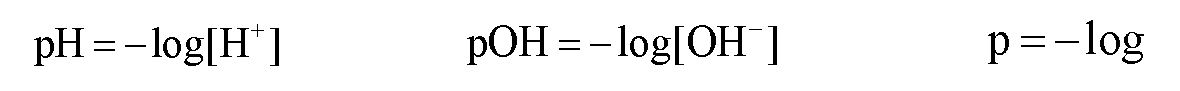Concept #2: The connection to Hydronium ion and Hydroxide ion concentrations.

If we know the pH or pOH concentration then we can determine the concentration of hydronium ions or hydroxide ions.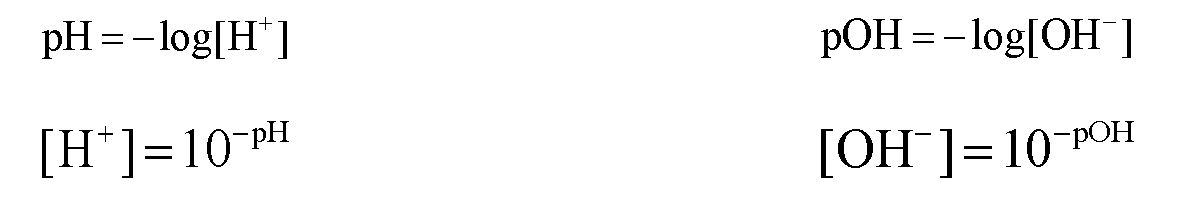Concept #3: The pH Scale.

If the pH is less than 7 then the solution is acidic, if the pH is equal to 7 then the solution is neutral and if the pH is greater than 7 then the solution is basic.

Concept #4: The pH and pOH connection.

Under normal conditions when the concentration is less than 1.0 M the pH scale is between 0 to 14. pH and pOH are connected by the following equation: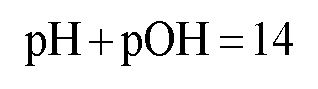Example #1: What is the hydroxide ion and hydronium ion concentration of an aqueous solution that has a pH equal to 6.12?

Practice: Which of the following solutions will have the lowest concentration of hydronium ions?

Practice: Which of the following statements about aqueous solutions is/are true?

Example #2: A solution is prepared by dissolving 0.235 mol Sr(OH)2 in water to produce a solution with a volume of 750 mL.

a) What is the [OH-]?

b) What is the [H+]?

Practice: What is the Kw of pure water at 20.0°C, if the pH is 7.083?

###### Auto – Ionization Product

Concept #5: Understanding the Auto-Ionization Reaction.

In a self-ionization reaction two water molecules react with one another, where one acts an acid and the other as a base.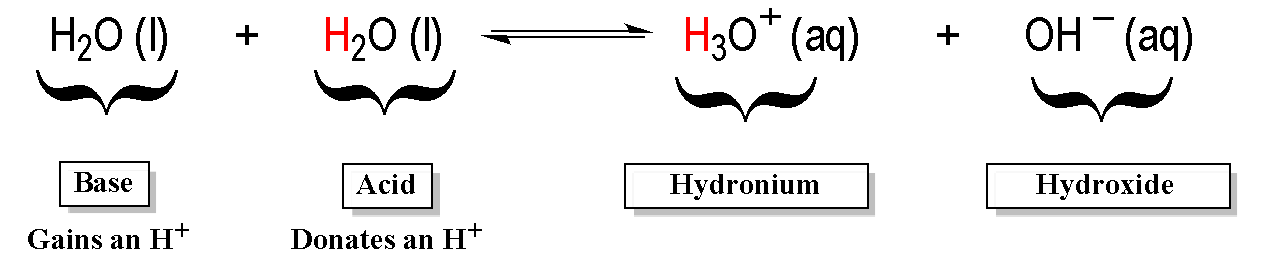The ionization product of Kw is used in this self-ionization equation and ignoring solids and liquids gives the  equilibrium equation as: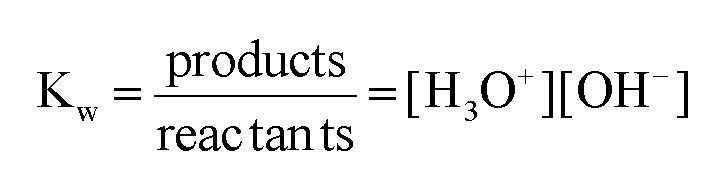Example #3: Determine the concentration of hydronium ions for a neutral solution at 25oC and at 50oC.

###### pH of Strong Acids and Bases

If we have a STRONG ACID or STRONG BASE then we DO NOT use an ICE chart to find the pH of solution because the yield of H+ and OH  ions are known.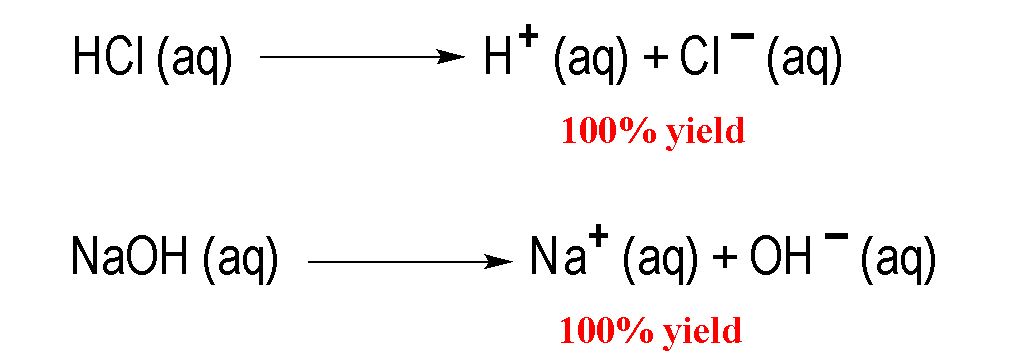Concept #6: Calculating the pH of a Strong Acid or Strong Base.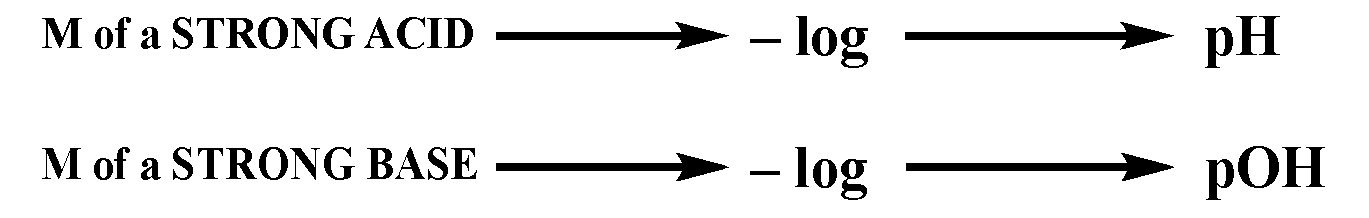Example #4: Calculate the pH of a 0.0782 M solution of CaH2.

Example #5: Calculate the pH of a 0.000550 M HBr solution to the correct number of significant figures.

1. 3.3
2. 3.26
3. 3.260
4. 3.2596
5. All are correct

Practice: Calculate the pH of 50.00 mL of 4.3 x 10-7 M H2SO4.

###### pH of Weak Acids and Bases

If we have a WEAK ACID or WEAK BASE then we DO use an ICE chart to find the pH of solution because the yield of H+ and OH  ions are unknown.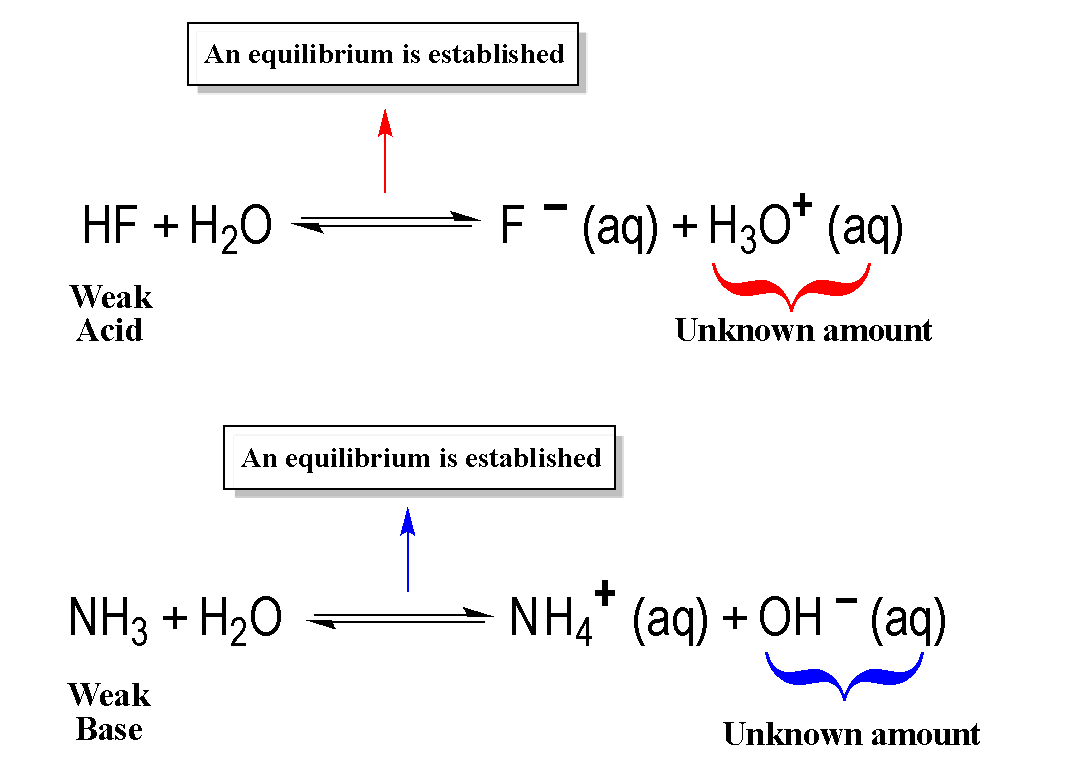Concept #7: Calculating the pH of a Weak Acid or Weak Base.

To determine the pH of a weak acid or weak base we must use an ICE Chart.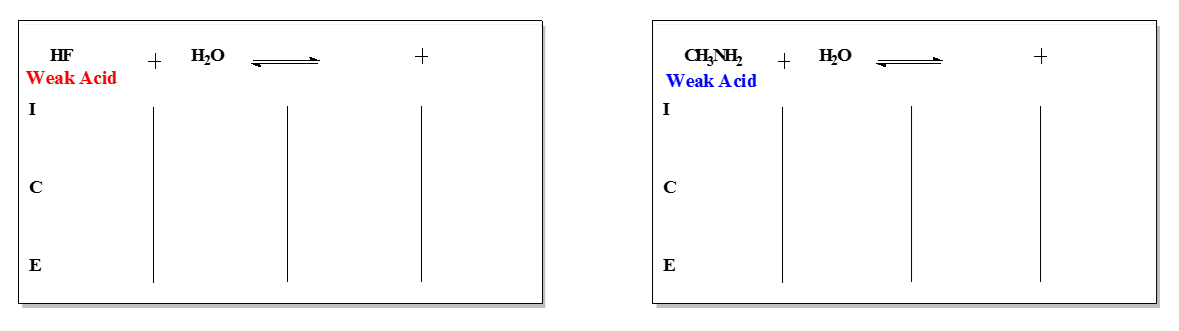Example #6: Pryridine, an organic molecule, is a very common weak base.

C5H5N (aq) + H2O (l)  ⇌  C5H5NH+ (aq) + OH- (g)

Assume you have a 0.0225 M aqueous solution of pyridine, C5H5N, determine its pH. The Kb value for the compound is 1.5 x 10-9

Example #7: An unknown weak base has an initial concentration of 0.750 M with a pH of 8.03. Calculate its equilibrium base constant.

Practice: Determine the pH of a solution made by dissolving 6.1 g of sodium cyanide, NaCN, in enough water to make a 500.0 mL of solution. (MW of NaCN = 49.01 g/mol). The Ka value of HCN is 4.9 x 10-10.